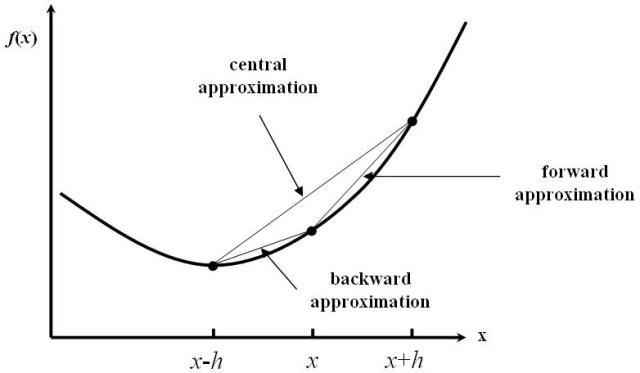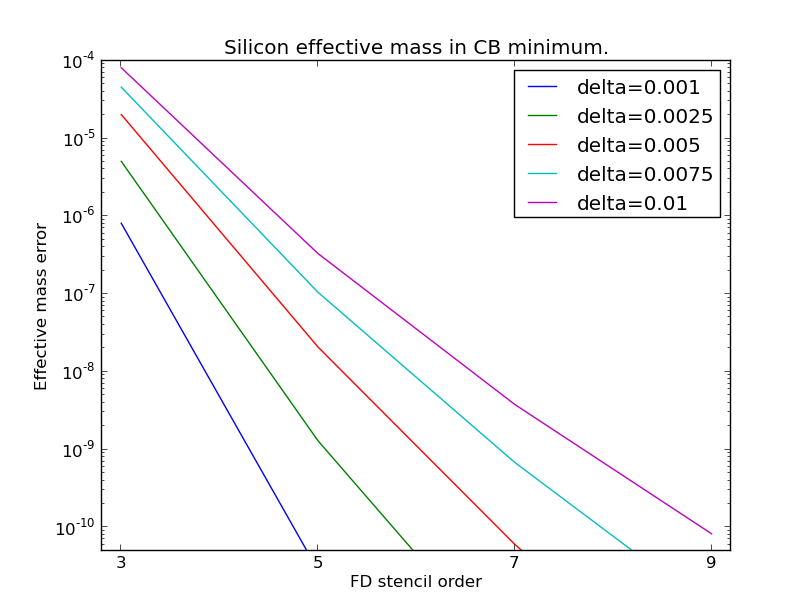# EffectiveMass¶

class EffectiveMass(configuration, symmetry_label=None, kpoint_cartesian=None, kpoint_fractional=None, bands_below_fermi_level=None, bands_above_fermi_level=None, band_index=None, stencil_order=None, delta=None, direction_cartesian=None, direction_fractional=None, method=None)

Constructor for the effective mass object.

Parameters: configuration (BulkConfiguration) – The bulk configuration with an attached calculator for which to calculate the effective mass. symmetry_label (str) – The k-point (as a symmetry point) at which to calculate the effective mass. This option is mutually exclusive to kpoint_cartesian and kpoint_fractional. Default: 'G' (Gamma-point) kpoint_cartesian (PhysicalQuantity of type inverse length) – The k-point (in Cartesian coordinates) at which to calculate the effective mass. This option is mutually exclusive to symmetry_label and kpoint_fractional. Default: [0.0, 0.0, 0.0] * Angstrom**-1 kpoint_fractional (list(3) of floats) – The k-point (in fractional coordinates) at which to calculate the effective mass. This option is mutually exclusive to kpoint_cartesian and symmetry_label. Default: [0.0, 0.0, 0.0] bands_below_fermi_level (int) – The number of bands below the Fermi level per principal spin channel to include. This option is mutually exclusive to band_index. Default: 1 bands_above_fermi_level (int) – The number of bands above the Fermi level per principal spin channel to include. This option is mutually exclusive to band_index. Default: 1 band_index (int) – The band index at which to calculate the effective mass. This option is mutually exclusive to bands_below_fermi_level and bands_above_fermi_level. stencil_order (int) – The number of points to use in the second order derivative stencil. Default: 5 delta (PhysicalQuantity of type inverse length) – The distance between neighboring points in the stencil. Default: 0.001 * Angstrom**-1 direction_cartesian (PhysicalQuantity of type inverse length) – Direction in Cartesian coordinates along which to calculate the effective mass. This option is mutually exclusive to direction_fractional. Default: [1, 0, 0] * Angstrom**-1 direction_fractional (list(3) of floats) – Direction in fractional coordinates along which to calculate the effective mass. This option is mutually exclusive to direction_cartesian. method (Numerical | Analytical) – The method for calculating the effective mass. The option Analytical is not supported by PlaneWaveCalculator. Default: Numerical
energies()
Returns: The band energies. PhysicalQuantity of type energy
evaluate(spin=None, band=None)

Return the effective mass.

Parameters: spin (Spin.Up | Spin.Down | Spin.All) – The spin for which to evaluate the effective mass. For non-collinear and spin-orbit calculations use Spin.All. Default: Spin.All band (int) – The band for which to evaluate the effective mass. Default: All bands The effective mass. PhysicalQuantity with the unit electron_mass
kPoint()
Returns: The k-point for which the effective mass was calculated. numpy.array
metatext()
Returns: The metatext of the object or None if no metatext is present. str | unicode | None
nlprint(stream=None)

Print a string containing an ASCII table useful for plotting the AnalysisSpin object.

Parameters: stream (python stream) – The stream the table should be written to. Default: NLPrintLogger()
setMetatext(metatext)

Set a given metatext string on the object.

Parameters: metatext (str | unicode | None) – The metatext string that should be set. A value of “None” can be given to remove the current metatext.

## Usage Examples¶

The effective mass of electrons and holes is related to the local curvature of the electronic band energy, $$E(k)$$:

$m_{\text{eff}} \propto \frac{1}{\frac{\partial^2 E(k)}{\partial k^2}}.$

A finite-difference (FD) representation of derivatives of $$E(k)$$ is used to compute the effective mass from band structures. The FD approximation for the local derivative of a function $$f(x)$$ is basically derived from a Taylor expansion of $$f(x)$$ around the central point, $$x$$. The n’th derivative is therefore approximated as a sum over function values multiplied by a weight:

$\frac{\partial^nf(X_0)}{\partial X^n} = f^{(0)} (X_0) \thickapprox \sum_{i} w_{i}^{(n)} f(x_1)/h^{2}$

The 5-point FD stencil uses function values in the five points $$x$$, $$x \pm h$$, and $$x \pm 2h$$ to approximate the second-order derivative, $$f''(x)$$. This is exact up to and including $$O(h^4)$$ contributions to the curvature (for $$h<1$$). Beyond that, accuracy improves as the spacing, $$h$$, gets smaller.Fig. 159 Finite-difference stencils: Numerical approximations for local derivatives of continuous functions.

The FD method is in general much more accurate than a simple parabolic fit to band energies. The QuantumATK default is the 5-point FD central stencil with a distance (delta) of 0.001 Å-1 between each point. This gives very accurate results in most cases. The FD accuracy can in principle be improved by increasing stencil_order and decreasing delta, but the latter should not be so small that numerical noise starts dominating the error. The figure below shows the error on computed effective masses for some realistic combinations of stencil_order and delta. For more information on 5-point stencils, see the relevant Wikipedia entry.Fig. 160 Errors in the silicon effective mass as a function of the number of points in the FD stencil. Plotted for different distances between the points.

Calculate the effective mass in the silicon conduction band minimum:

# -------------------------------------------------------------
# Bulk configuration
# -------------------------------------------------------------

# Set up lattice
lattice = FaceCenteredCubic(5.4306*Angstrom)

# Define elements
elements = [Silicon, Silicon]

# Define coordinates
fractional_coordinates = [[ 0.  ,  0.  ,  0.  ],
[ 0.25,  0.25,  0.25]]

# Set up configuration
bulk_configuration = BulkConfiguration(
bravais_lattice=lattice,
elements=elements,
fractional_coordinates=fractional_coordinates
)

# -------------------------------------------------------------
# Calculator
# -------------------------------------------------------------
numerical_accuracy_parameters = NumericalAccuracyParameters(
k_point_sampling=(5, 5, 5),
density_mesh_cutoff=25.0*Hartree,
)

calculator = LCAOCalculator(
numerical_accuracy_parameters=numerical_accuracy_parameters,
)

bulk_configuration.setCalculator(calculator)
nlprint(bulk_configuration)
bulk_configuration.update()

# -------------------------------------------------------------
# Effective mass
# -------------------------------------------------------------
effective_mass = EffectiveMass(
configuration=bulk_configuration,
kpoint_fractional=[0.425000, 0.000000, 0.425000],
bands_below_fermi_level=1,
bands_above_fermi_level=1,
stencil_order=5,
delta=0.001000*Angstrom**-1,
)

effective_mass.nlprint()


si_effective_mass.py